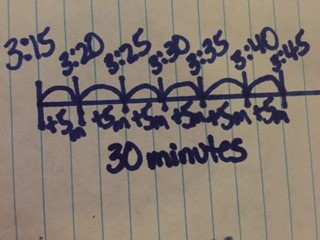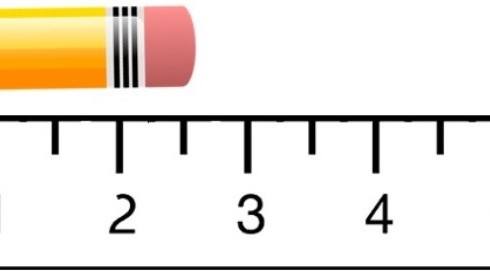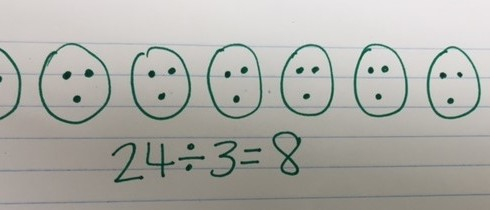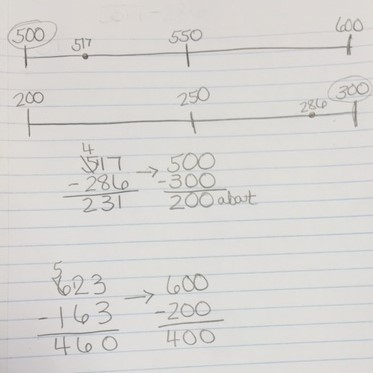## ArchivesTo solve story problems involving elapsed time, encourage students to draw open number lines to help students conceptually understand the concept. This could prevent common misconceptions students may have when determining elapsed time. Make sure to include different types of…Telling a time is an important concept for everyday life. It’s also one that students some time struggle with if they don’t understand how a clock works. In 3rd grade, students are expected to: • Tell and write time to…Students in 3rd grade are expected to measure length to the nearest whole inch, half inch and quarter inch. In order for students to be successful in measuring length and representing that length as fractions, consider using paper rulers to…Students in 3rd grade are expected to measure length to the nearest whole inch, half inch and quarter inch. (See the item specifications listed below). It is helpful to begin this learning by relating one whole inch to one whole…In 3rd grade, students are expected to generate simple equivalent fractions. Example: 1/2 = 4/8 and 4/6 = 2/3. Models should be used to help students identify equivalent fractions. Sentence strips or strips of paper cut into congruent rectangles can…Check out the video below for a quick and easy way to reinforce mathematics concepts on a number line.In 3rd grade students are expected to, “Understand a fraction as the quantity formed by 1 part when a whole is partitioned into equal parts.” The best way for students to develop this understanding is by putting fractions into real…Modeling Fractions on a Number Line! In 3rd grade students are expected to, “Understand a fraction as a number on the number line; represent fractions on a number line.” This is a concept that students struggle with if you don’t…In 3rd grade students are developing understanding of fractions. Previously in 1st & 2nd grade they have decomposed shapes into equal parts and described them using fraction language, but in 3rd grade is the first year they represent the fractions…In 4th grade students also use rounding to estimate sums and differences. This strategy is used to make sure that their estimation is reasonable. They used this strategy in 3rd grade. Below is some examples of estimation that students learned…
In 4th grade students use strategies to estimate numbers. One strategy for estimation is rounding. This is built off of the foundation they received in 3rd grade. Below is how students learned how to use number lines to estimate numbers…